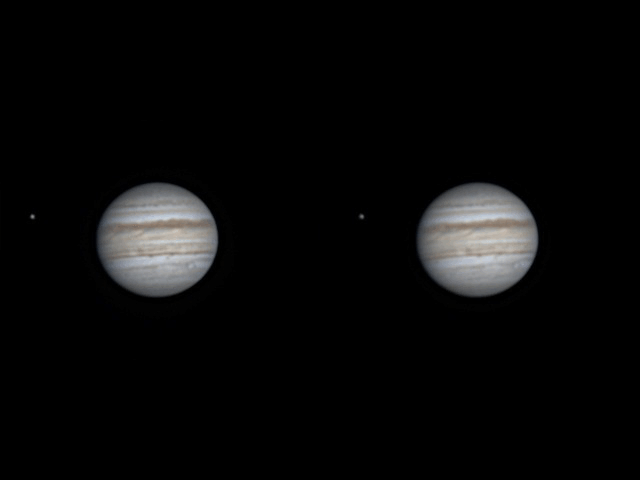ASI 224MC (cooled), TSO ADC, N250/1200, HEQ5, home observatory, mountpusherThe cross eye 3D – this is an illusion actually. Since the planet rotates and the moon orbits, applying a small time offset will give the illusion of a different perspective, and due to the orbital vs rotational speed difference, the moon will appear to be in front or back, given the directionality. Hwoever, since a satellite’s shadow moves together with the moon and not the planet, a shadow pops out of Jupiter and ruins the 3D effect. In case of a perspective shift, the shadow would stay in the same spot relative to the clouds.

The individual frames:

szurs

```<?php

function harom(\$n){
\$n = ''.\$n;
while (strlen(\$n) < 3){
\$n = '0'.\$n;
}
return \$n;
}

foreach (glob('output/*.jpg') as \$old_output){
}

\$input_files = glob('input/*.jpg');
\$delta = 4;

for (\$q=\$delta; \$q<count(\$input_files) - \$delta; \$q++){
\$left = imagecreatefromstring(file_get_contents(\$input_files[\$q-\$delta]));
\$right = imagecreatefromstring(file_get_contents(\$input_files[\$q]));

\$out = imagecreatetruecolor(imagesx(\$left) + imagesx(\$right), imagesy(\$left)+imagesy(\$right));
imagecopy(\$out, \$left, 0, round(imagesy(\$left) / 2), 0, 0, imagesx(\$left),imagesy(\$left));
imagecopy(\$out, \$right, imagesx(\$left), round(imagesy(\$left) / 2), 0, 0, imagesx(\$left),imagesy(\$left));
imagejpeg(\$out, 'output/output_'.harom(\$q).'.jpg', 100);
imagedestroy(\$out);
imagedestroy(\$left);
imagedestroy(\$right);
}

?>```